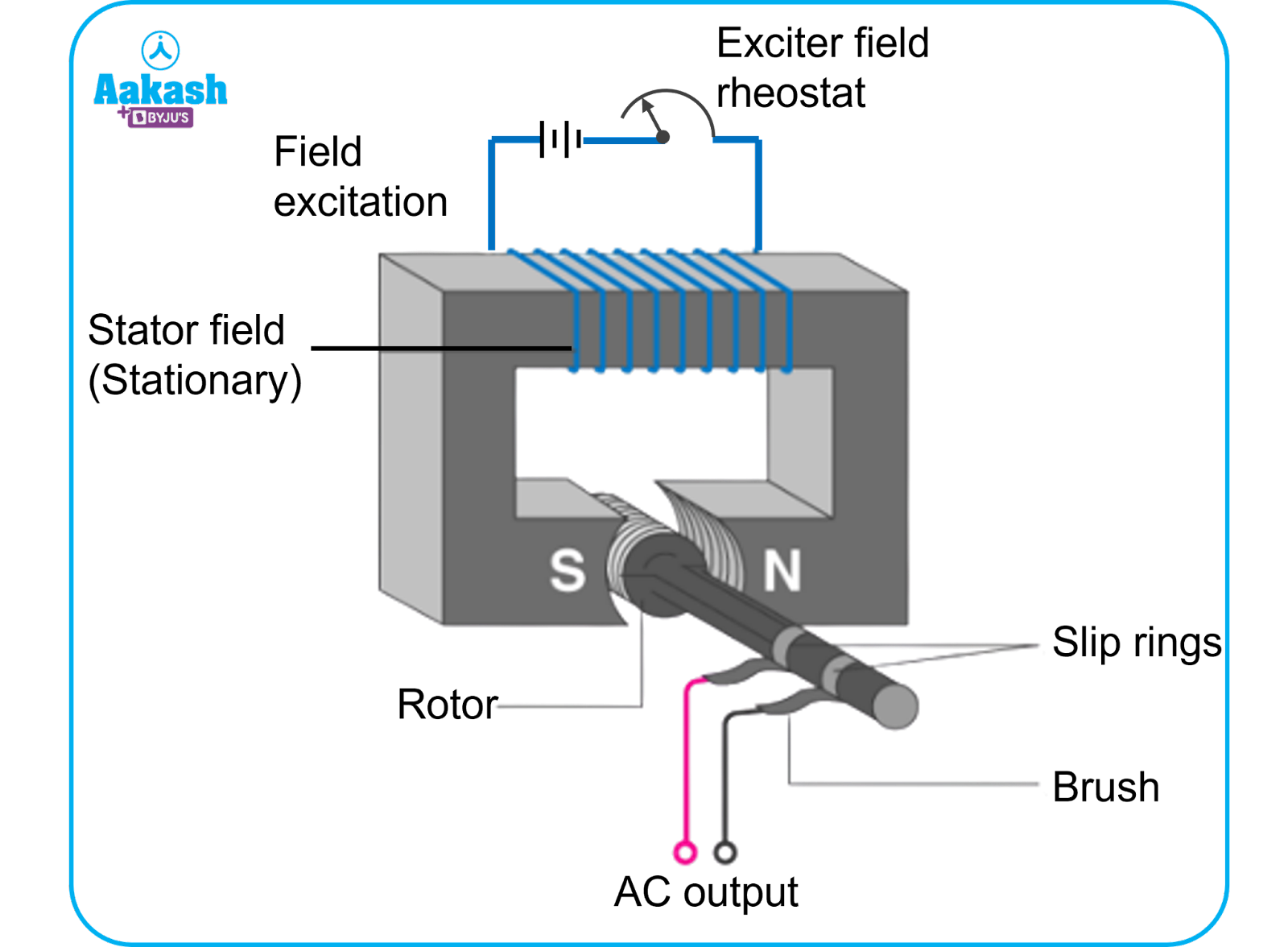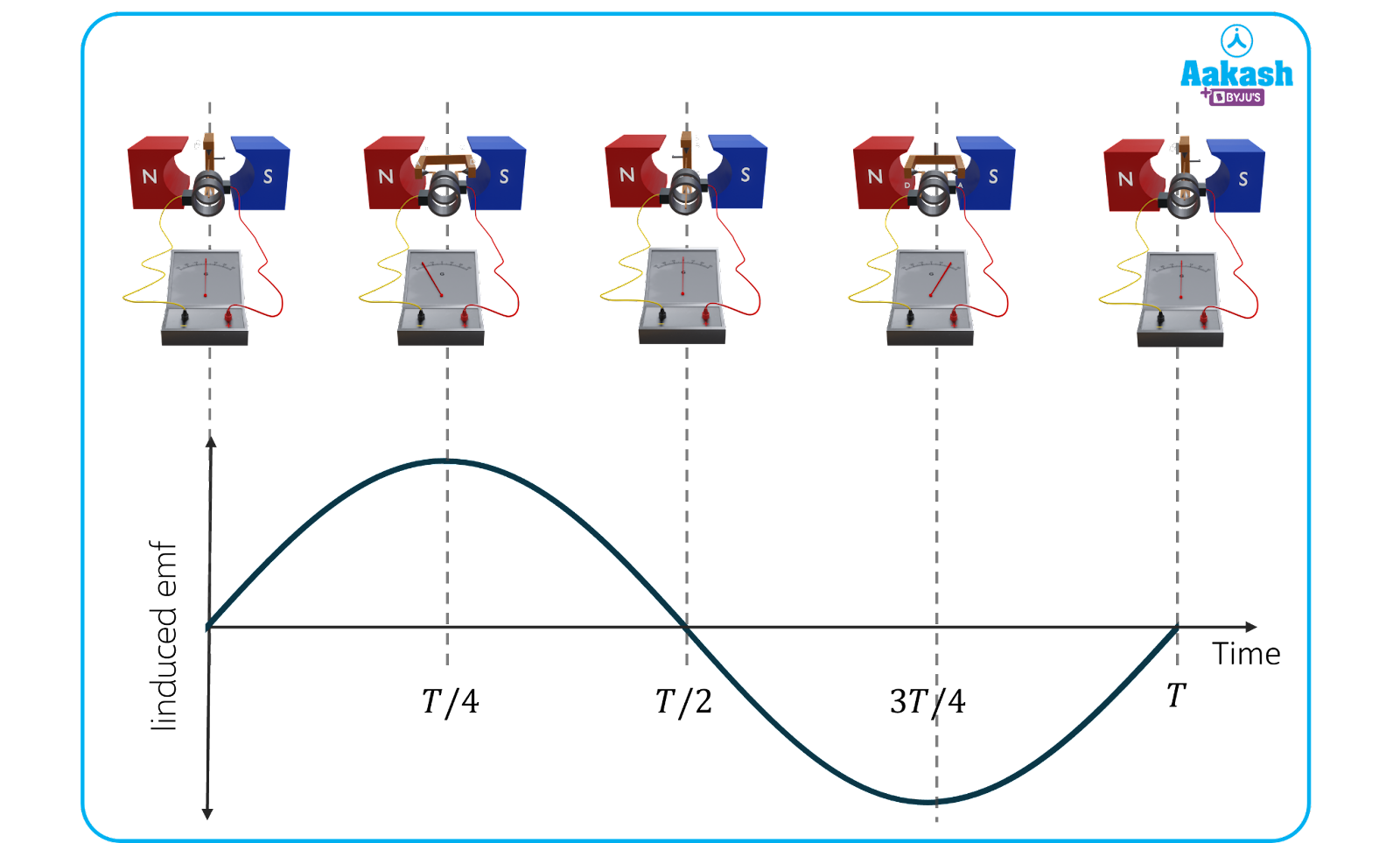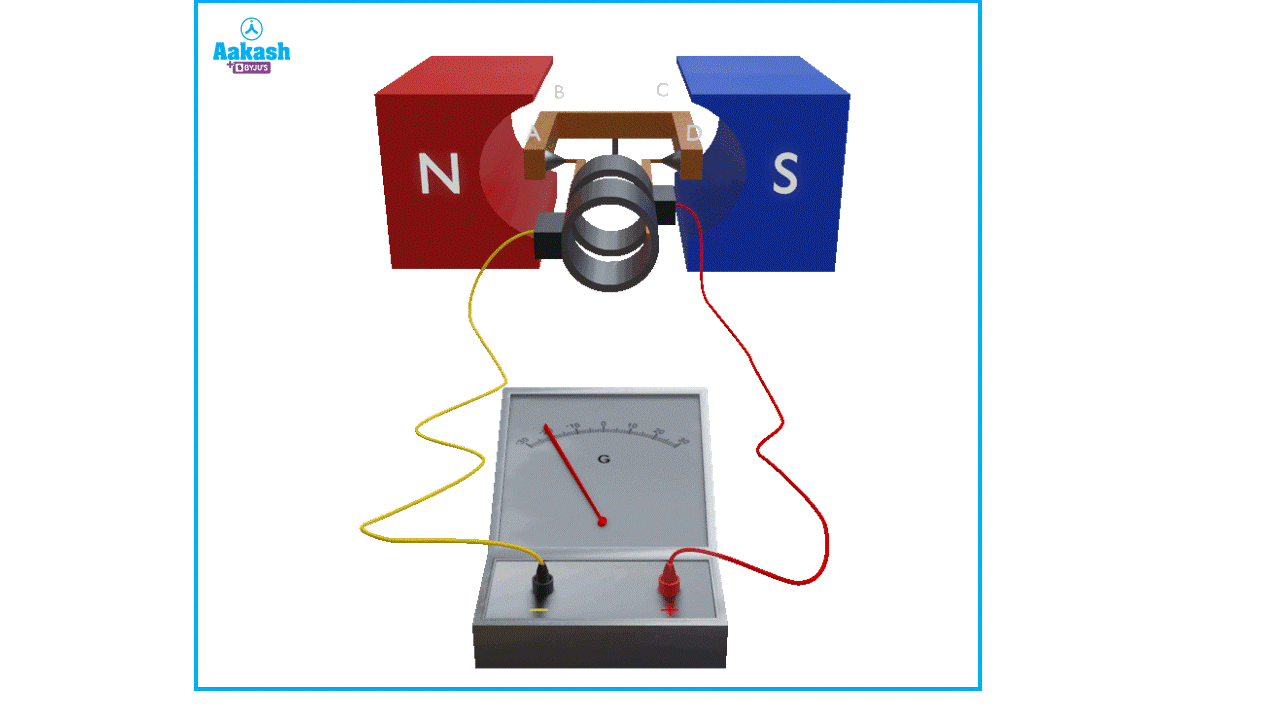• Call Now

1800-102-2727•

# AC generator - principle, construction, applications, practice problems, FAQs

Sourav runs a small restaurant in a village where power cuts happen frequently. Customers who came to eat there never left satisfied; thanks to lack of proper supply, the fans, lights and mixers in Sourav’s restaurant were never functioning smoothly. One day, he notices that the shopkeeper next to him had his lights on while his restaurant had no power. Sourav starts to wonder how this is possible; so he asks the shopkeeper. He said “ I have a generator, I don’t have to worry about power cuts”. Today, workplaces, schools, hostels, run on generators which supply electricity, so that they don’t have to face power down issues. Before the advent of the generators, electricity was generated using dynamo, which provided DC electricity. But as time progressed, scientist and inventor Charles F. Brush constructed an electric arc lamp which supplied a constant load current of high magnitude, which led him to invent the AC generator. In this article, we will explore AC generators in detail.

• Definition of a generator
• Principle of a generator
• Construction of an AC generator
• Working of an AC generator
• Practice problems
• FAQs

## Definition of a generator

A generator is a device which converts mechanical energy into electrical energy. The input mechanical energy needed for the generator to function is supplied by engines or turbines run by compressed natural gas (CNG) or diesel or the mechanical energy in the flowing water in a hydel power plant. An AC generator produces alternating current.

## Principle of a generator

A generator works on the principle of Faraday’s laws of electromagnetic induction; i.e when a coil is rotated in a magnetic field, a magnetic flux change is produced in the coil, as a result, an emf or electromotive force is produced in it. This in turn produces an induced current in the coil. Magnetic flux can be varied either by varying the magnetic field or by rotating the coil in a steady magnetic field. The magnitude of the induced current produced depends upon the strength of the magnetic field, number of turns in the armature, the area of cross section of the coil and the speed with which it is rotated.

## Construction of an AC generator

A typical AC generator has the following parts:

1) Magnetic field

2) Armature

3) Rotor

4) Stator

5) Slip rings

6) Carbon brushes1) Magnetic field

The necessary magnetic field is provided by permanent horseshoe magnets; which have North south polarities and supply a strong magnetic field.

2) Armature

Armature is a coil with many turns wound over a soft-iron core. The latter provides necessary support for the armature. The axis of rotation of the coil is perpendicular to the magnetic field.

3) Rotor

The rotating component of a generator is termed as its rotor.

4) Stator

Stator is the stationary part of a generator which does not move. It is laminated to protect against eddy current losses.

5) Slip rings

Slip rings are used to transmit electrical energy from the stator to the rotor.

6) Brushes

Carbon brushes made of graphite permanently touch the slip rings. They are connected to the external circuit.

## Working of an AC generator

When the armature coil rotates, the flux lined with it can be written as =NBA cos t

where,

N-number of turns in the coil
B- Magnetic field due to permanent magnets
A- Area of cross section of the coil
- Angular velocity
t- instantaneous time lapsed

$e=-\frac{d\varphi }{dt}=-\frac{d\left(NBAcos\omega t\right)}{dt}=NBA\omega sin\omega t$

$e={e}_{0}sin\omega t--\left(i\right)$

e0=NBA indicates the maximum value of emf.

Equation (i) shows the induced emf varies sinusoidally with time.

The maximum value of induced current ${I}_{o}=\frac{NBA\omega }{R}$

Where R indicates the resistance of the armature coil.

Now $\omega =\frac{2\pi }{T}$ where T indicates the time period of the coil in seconds. It is the time taken for one full rotation.From (i), we can write,

$e={e}_{0}sin\left(\frac{2\pi }{T}\right)t--\left(ii\right)$

When …. the value of emf induced is minimum (e=0). This happens when the normal drawn to the armature coil, while rotating, cuts the magnetic field in a parallel direction.Fig showing position of the armature when maximum emf is induced

When t=T/4,3 T/4 ,5T/4…. the value of emf induced is maximum.(e=e0)

This happens when the normal of the armature coil, while rotating, cuts the magnetic field at right angles.

## Practice problems

Q. In a region where a uniform magnetic field, B=10-2 T exists, a circular coil of radius 30 cm and resistance 2 is made to rotate about an axis which is perpendicular to the magnetic field B. If the speed of rotation of the coil is 200 rpm, the amplitude of the alternating current induced in the coil is

(a) 22 mA (b) 30 mA (c) 6 mA (d) 200 mA

A. c

Given B=10-2 T

Area of the coil $A=\pi {\left(0.3\right)}^{2}$

$\omega =2\pi ×\left(\frac{200}{60}\right)=\frac{20\pi }{3}$

Number of turns,

The maximum value of induced current${I}_{0}=\frac{NBA\omega }{R}$

Q. A square loop of side 10 cm and resistance 0.5 is used in an AC generator. The time varying magnetic field 0.70 T/ s exists between the poles. What is the maximum current produced in the coil during this interval?

(a) 10 mA (b) 12 mA (c) 14 mA (d) 16 mA

A. c

Change in magnetic field

Area of the coil A=0.10.1=10-2 m2

Resistance R=0.5

Let

Q. The current flowing in the armature of a coil varies with time according to the equation I=10 sin(100t)where t is in seconds and I in ampere. Calculate the time at which maximum current will be induced in the coil.

(a) 5 milliseconds (b) 3 milliseconds (c) 1 milliseconds (d) 18 milliseconds

A. a

Given, I=10 sin(100t)

The above expression is maximum when $sin\left(100\pi t\right)=1⇒100\pi t=\frac{\pi }{2}$

$t=\frac{1}{200}=5$ milliseconds.

Q. The magnetic field in an armature coil varies with time according to the relation B=B0t2, B0 is a +ve constant. If r indicates the radius of the coil, find the emf induced in the coil at t=1 s.

(a) $\pi {r}^{2}{B}_{0}$(b) $2\pi {r}^{2}{B}_{0}$ (c) (d)None of these

A.b

Given $B={B}_{0}{t}^{2}⇒\frac{dB}{dt}={2B}_{0}t$

## FAQs

Q. What is the principle of an AC generator?
A.
When a coil is rotated in a magnetic field, a magnetic flux change is produced in the coil, as a result, an emf or electromotive force is produced in it.

Q. What is the difference between AC generator and DC generator?
A.
A DC generator produces DC current, which is unidirectional in nature; its direction does not vary with time. AC generator produces Alternating current, direction of which varies with time. It is as if the power source is changing its poles continuously.

Q. Write two applications of AC generators.
A.
AC generators are used in areas where power cuts are frequent. Also, power plants make use of AC generators.

Q.Write one advantage of AC generator over DC generator.
A.
Design of the AC generator is simple compared to the DC generator, because AC generator does not need a commutator while DC generator does.Talk to our expert
Resend OTP Timer =
By submitting up, I agree to receive all the Whatsapp communication on my registered number and Aakash terms and conditions and privacy policy